Forbidding chains for absolute beginners

What's that ?

This concept generalizes all known examples of sudoku-solving tips and keeps applying when known tips can do no more.

The closest concept I found by googling around was "forcing chains" but I think this is a little more general pattern. Although it's true that forbidding chains cannot solve every puzzle, very few resist ! (for example, none from sudoku.com.au ...)

I First examples.

Example 1.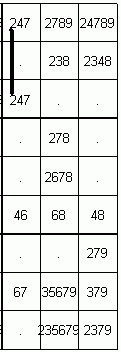In that diagram is drawn a thick line joining the possible 2s in a7 and a9. It means : "at least one of assumptions (a7=2), (a9=2) is true" (check it's so : there must be a 2 somewhere in column a). From now, this will be noted (a7=2)==(a9=2) : (a7=2)==(a9=2) means : "at least one of assumptions (a7=2), (a9=2) is true"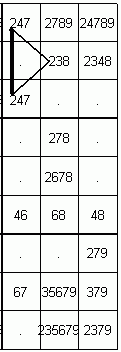The thin line joining the possible 2s in a9,b8 means : "at most one of assumptions (a9=2), (b8=2) is true" (check it's so : there must not be two 2s in block Bb8). From now this will be noted (a9=2)--(b8=2) : (a9=2)--(b8=2) means "at most one of assumptions (a9=2), (b8=2) is true" Of course the other link indicated in diagram : (b8=2)--(a7=2) is justified the same way.

Now as experienced sudokuers, you know well that (b8=2) is no more possible. The logical reason may sound like "if b8=2, then no more 2s in Bb8 : impossible because there must be a 2 in column a" or "Look at only two possibles (a7=2), (a9=2) in column a : in both cases we have no more b8=2 since there is already a 2 in block Bb8", it doesn't matter ; fortunately you integrated that rule long ago and can clean your grid by saying more shortly :

"Only two possible (a7=2),(a9=2) in column Ca forbid b8=2 (and samely forbid b9=2, c8=2, c9=2)"

that I abbreviate just a little bit more in

"(a7=2)==(a9=2) forbids b8=2 (and samely forbids b9=2, c8=2, c9=2)"

and to remind the geometric aspect of diagram, I call it a triangular situation.

At this point, it's sane to think : "What a complicate point of view for something every sudokuer understands so easily" ! Agree, but wait... It's a "for absolute beginners" page.

Example 2.

Kindly provided by Philippe Lacourt-Gayet :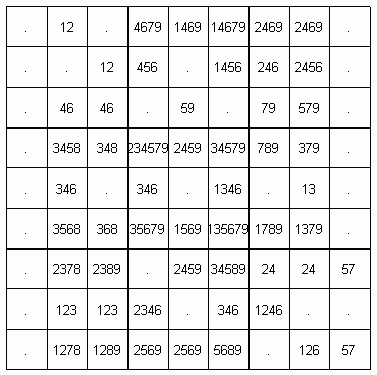Can you clearly see here that we have no more c2=1? (if you can this page is not for you, good bye...)

Still here? so look beyond :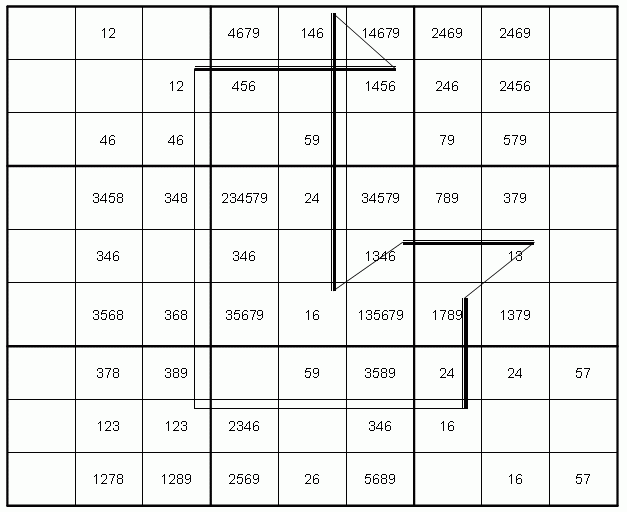Looking at possible 1s in this diagram, check the correctness of indicated (thick/thin) links : (c8=1)==(f8=1) : there must be a 1 in R8 ; (f8=1)--(e9=1) : there must not be two 1s in Be8 ; (e9=1)==(e4=1) : there must be a 1 in Ce ; (e4=1)--(f5=1) : there must not be two 1s in Be5 ; (f5=1)==(h5=1) : there must be a 1 in R5 ; (h5=1)--(g4=1) : there must not be two 1s in Bh5 ; (g4=1)==(g2=1) : there must be a 1 in Cg. Now let's say : (c8=1) is either true or false ; but in the second case : f8=1 (guess why ? because it's thick linked to c8=1), e9 is not 1 (thinlinked to f8=1), e4=1 (check why), f5 is not 1 (check why), h5=1 (check why), g4 is not 1 (check why), g2=1 (check why). In short , one at least between assumptions (c8=1), (g2=1) is true, which is noted (c8=1)==(g2=1).

Can you NOW clearly see why we have no more c2=1? It comes of course from the last two links we didn't use : (c2=1) is thinlinked both to (c8=1) and (g2)=1 ! I leave the conclusion to you. As for me, I summarize all this in :

(c8=1)==(f8=1)--(e9=1)==(e4=1)--(f5=1)==(h5=1)--(g4=1)==(g2=1) forbids c2=1

and to remind the geometric aspect of diagram, I call it a nonagonal situation.

II The logical background

In this section we deal with assumptions (these are sentences that may be true or false) noted A,B,C...

1) the definitions

Definition 1 : Let A, B be two assumptions. We say that A,B are thicklinked and note A == B when at least one of A,B is true . We say that A,B are thinlinked and note A--B when at most one of A,B is true.

In other words :
a) By definition, when one edge of a thicklink is false, then the other is true.
b) By definition, when one edge of a thinlink is true, then the other is false.

2) The triangle theorem

Now we state the forbidding power of a thick link. Imagine such a thick link closed in a triangle by two thin links :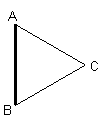so that : A==B, ie one at least of A,B is true ; C--B, ie one at most of B,C is true ; C--A, ie one at most of C,A is true. We can now argue : "What if C is true? then A and B are false (since C--A and C--B) : impossible since A==B. So C is false." We can also argue : "if C is true then (by C--A) A is false, so (by A==B) B is true, and (by B--C) C is false ! So C is false..." Or still : "since A==B we have either A or B. In first case : C is false because A--C. In second case : C is false because B--C. In both cases C is false."

No matter how we argue it, we just have established a theorem of logics (a loud trumpet blow would sound great here) :

Theorem 1 : when three assumptions A,B,C verify C--A == B--C, then C is false.

In other words :

Theorem 1 (restated) : when A and B are thicklinked, any assumption thinlinked to both A and B is false.

3) longer chains.

Here we concentrate on the logical scheme A==B--C==D. We can argue that : if A is false then B is true (because A==B) so C is false (because B--C) and D is true (because C==D). In other words : A==D, so that (trumpets again, please) :

Theorem 2 : when assumptions A,B,C,D verify A==B--C==D, then A==D.

We will say that A==B--C==D is a forbidding chain (of length 4), since A==D and by theorem 1, any assumption thinlinked to both A and D is false.

Theorem 2 (restated) : when assumptions A,B,C,D verify A == B--C == D, any assumption thinlinked to both A and D is false.

But now nothing can stop forbidding chains to grow at any (even) length : if for instance we have A==B--C==D--E==F, then theorem 2 allows to shorten it in A==D--E==F, and again theorem 2 gives A==F. More generally, think a bit and you'll be convinced that :

Theorem 3 : when assumptions A,B,C,...X,Y,Z verify A==B--C...X--W==Z, where thick and thin links alternate, then A==Z.

or once more :

Theorem 3 (restated) : when assumptions A,B,C,...X,Y,Z verify A==B--C...X--W==Z, where thick and thin links alternate , then any assumption thinlinked to both A and Z is false.

4) In short.

III Forbidding-chain explanation of usual solving tips

In fact, every usual solving tip appears as a simple special case of forbidding chain.

1) naked pairs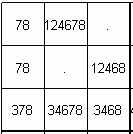Check that (a2=7)==(a2=8)--(a3=8)==(a3=7), which forbids b1=7,b3=7. Check that (a2=8)==(a2=7)--(a3=7)==(a3=8), which forbids b1=8, b3=8, c1=8,c2=8. This is the "naked pair" pattern, seen as special case of forbidding chains.

2) hidden pairsCheck that (b3=1)==(c2=1)--(c2=2)==(b3=2), which forbids b3=4,6,7,8. Check that (c2=1)==(b3=1)--(b3=2)==(c2=2), which forbids c2=4,6,8. This is the "hidden pair" pattern.

3) Xwing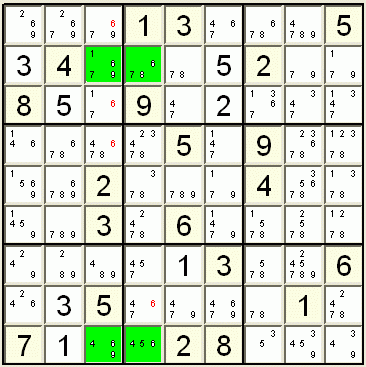Here is a basic example, with the 6 in c1d1c8d8 : Check that the chain (c1=6)==(d1=6)--(d8=6)==(c8=6) forbids c5,c7,c9=6. Check that the chain (d1=6)==(c1=6)--(c8=6)==(d8=6) forbids d2=6. This is the X-wing pattern seen as special case of forbidding chains.

4)XY wing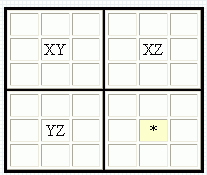I've found this diagram here, a webpage explaining the XYwing concept. Page says : It can be easily seen that whichever value is in XY, the cell marked with the asterisk cannot be Z. It's nothing but the chain (b2=Z)==(b2=Y)--(b5=Y)==(b5=X)--(e5=Y)==(e5=Z) forbidding e2=Z.

5)Swordfish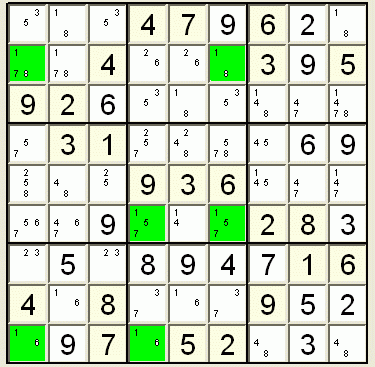This diagram comes from here, a webpage explaining the swordfish concept. Saying : "here is a swordfish with the 1s". It's nothing but the chain (d4=1)==(d1=1)--(a1=1)==(a8=1)--(f8=1)==(f4=1) that forbids e4=1. Note also that samely (f8=1)==(f4=1)--(d4=1)==(d1=1)--(a1=1)==(a8=1) forbids b8=1.